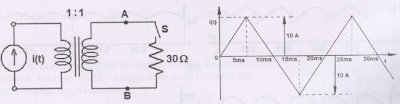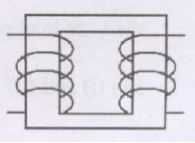## Friday, November 23, 2012

### Transformers Objective Questions: Part-3

Transformers Solved Objective Questions from Competitive Exams(Gate, IES)
Each Question Carries Two Marks:
Common Data for Questions 1 and 2: [GATE 2009]
The star-delta transformer shown above is excited on the star side with a balanced,4-wire,3-phase,sinusoidal voltage supply of rated magnitude. The transformer is under no load condition.With both S1 and S2 open, the core flux waveform will be [GATE 2009]
A. A sinusoidal at fundamental frequency
B. Flat-topped with third harmonic
C. Peaky with third-harmonic
D. None of these

 With S2 closed and S1 open, the current waveform in the delta winding will be  [GATE 2009]
A. A sinusoidal at fundamental frequency
B. Flat-topped with third harmonic
C. Only third-harmonic
D. None of these

Common Data for Questions 3 and 4:
The circuit diagram shows a two-winding, losses transformer with no leakage flux, excited from a current source,i(t),whose waveform is also shown. The transformer has a magnetizing inductance of 400/ πmH.The peak voltage across A and B, with S open is [GATE 2009]
A. 400/ πV
B. 800 πV
C. 4000/π V
D. 800/ πV

 If the waveform of i(t) is changed to i(t)= 10 sin(100πt)A, the peak voltage across A and B with S closed is [GATE 2009]
A. 400V
B. 240V
C. 320V
D. 160VThe figure above shows coils 1 and 2, with dot markings as shown, having 4000 and 6000 turns respectively. Both the coils have a rated current of 25A. Coil 1 is excited with single phase, 400V, 50Hz supply.

 The coils are to be connected to obtain a single phase, 400/1000V, auto-transformer to drive a load of 10kVA. Which of the options given should be exercised to realize the required auto-transformer? [GATE 2009]

A. Connect A and D; Common B
B. Connect B and D; Common C
C. Connect A and C; Common B
D. Connect A and C; Common D

 In the autotransformer obtained in Question 57, the current in each coil is [GATE 2009]
A. Coil-1 is 25A and Coil-2 is 10A
B. Coil-1 is 10A and Coil-2 is 25A
C. Coil-1 is 10A and Coil-2 is 15A
D. Coil-1 is 15A and Coil-2 is 10A

The single phase, 50Hz iron core transformer in the circuit has both the vertical arms of cross sectional area 20cm2 and both the horizontal arms of cross sectional area 10cm2. If the two windings shown were wound instead on opposite horizontal arms, the mutual inductance will [GATE 2009]A. Double
B. Remain same
C. Be halved
D. Become one quarter

Solution Hint:
Vertical arms A = 20cm2
Horizontal arms A = 10cm2
Wound in the horizontal arms means, Area reduces from 20cm2 to 10cm2.... So Inductance will be halved.

1.2.3.4.